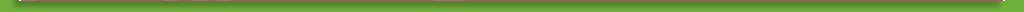This website requires Javascript to function properly.

# Welcome

Benford’s Law

Have your mind blown by these math facts.

Did you know? Well, probably not—we haven’t told you any of these fun math facts yet! Even if you do know some of them, there might be a few that you can share with your class and friends. Keep reading to learn the best math facts around!

1. In a room with 23 people, there is a 50% chance two of them have the same birthday! It’s called the birthday paradox. It works because you’re not comparing one person to the other 22, but all 23 to each other.2. Temperature-wise, -40˚C is the same as -40˚F. The formula for converting between the two temperature scales is (X˚F – 32) x 5/9 = X˚C. The formula just happens to match up at -40.
3. Ever hear someone say they’ll be there in a jiffy? A jiffy is a real measurement of time: it is 1/100th of a second.
4. The symbol for division, ÷, is called an obelus.
5. Math has been around since 30,000 BCE, according to markings found on animal bones.
6. A baseball diamond is a perfect rhombus—a parallelogram with opposite equal acute angles, opposite equal obtuse angles, and four equal sides.
7. A snail’s shell follows the Fibonacci sequence, where the two previous numbers are added together to get the next number.
8. Sugar cookies are tasty math. A perfect ratio of 3:2:1. Three parts flour, two parts fat, one part sugar.
9. Eratosthenes was an Egyptian mathematician who first calculated the circumference of Earth in the year 240 BCE. He was only 2% off.
10. The US Navy was once put out of action by dividing by zero. Or at least one ship was out of action. A zero was mistakenly entered into the USS Yorktown’s computer and the ship was stranded for two hours until the system could be reset.
11. A standard sheet of paper folded 103 times would result in a piece as thick as the observable universe.
12. The numbers on opposite sides of a standard die in a pair of dice add up to 7. If your die don’t do that, you should get a new one.
13. 111,111,111 x 111,111,111 = 12,345,678,987,654,321. Cool.
14. Zero is technically an even number.
15. A googolplex is such a large number, there isn’t enough room in the known universe to write it out in long-form.
16. Four is the only number that has the same amount of letters as the number.
17. The first two decimals of Pi spell pie backward. 3.14/PIE. Hungry yet?

Well, there are 17 fun facts you might not have known about numbers! Math might not always seem fun, but there is always a way to make it fun.

# Poll

On Thanksgiving will you...